GeeksforGeeks App
Open AppBrowser
Continue

## Related Articles

• RD Sharma Class 9 Solutions

# Class 9 RD Sharma Solutions – Chapter 8 Introduction to Lines and Angles- Exercise 8.4 | Set 3

### Question 21. In the given figure, transversal l intersects two linesmandn, ∠4 = 110° and ∠7 = 65°. Ism||n?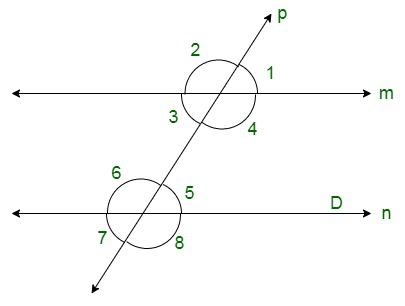Solution:

The figure is given as follows:

It is given that l is a transversal to lines m and n. Also,

∠4 = 110° and ∠7 = 65°

We need to check whether m || n or not.

We have ∠7 = 65°.

Also, ∠7 and ∠5 are vertically opposite angles, thus, these two must be equal. That is,

∠5 = 65° ………(i)

Also, ∠4 = 110°.

Adding this equation to (i), we get:

∠4 + ∠5 = 110° + 65°

∠4 + ∠5 = 175°

But these are the consecutive interior angles which are not supplementary.

Theorem states: If a transversal intersects two lines in such a way that a pair of consecutive interior angles is supplementary, then the two lines are parallel.

Thus, m is not parallel to n.

### Question 22. Which pair of lines in the given figure are parallel? Given reasons.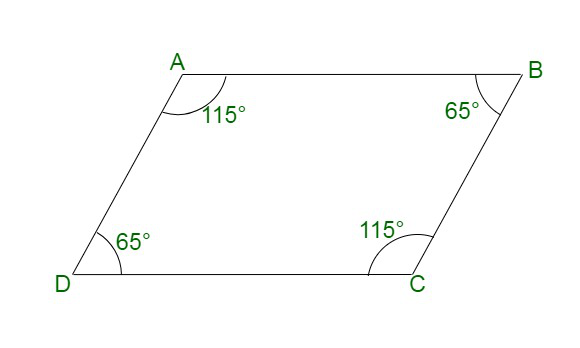Solution:

The figure is given as follows:

We have ∠BCD = 115° and ∠ADC = 65°.

Clearly,

∠BCD + ∠ADC = 115° + 65°

These are the pair of consecutive interior angles.

Theorem states: If a transversal intersects two lines in such a way that a pair of consecutive interior angles is supplementary, then the two lines are parallel.

Similarly, we have ∠DAB = 115° and ∠ADC = 65°.

Clearly,

∠DAB + ∠ADC = 115° + 65°

These are the pair of consecutive interior angles.

Theorem states: If a transversal intersects two lines in such a way that a pair of consecutive interior angles is supplementary, then the two lines are parallel.

Thus, AB || CD.

Hence, the lines which are parallel are as follows:

AD || BC and AB || CD.

### Question 23. If l, m, n are three lines such that l||m and n⊥l. Prove that n⊥m.

Solution:

The figure can be drawn as follows: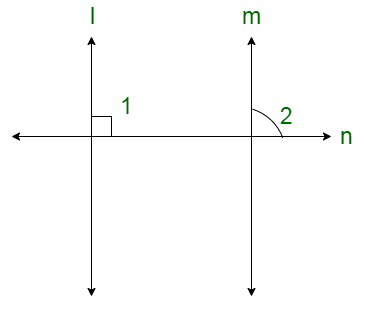Here, l || m and n ⊥ l

We need to prove that n ⊥ m.

It is given that n ⊥ l, therefore,

∠1 = 90° ……(i)

We have l || m, thus, ∠1 and ∠2 are the corresponding angles. Therefore, these must be equal. That is,

∠1 = ∠2

From equation (i), we get:

∠2 = 90°

Therefore, n ⊥ m.

Hence, proved.

### Question 24. In the given figure, arms BA and BC of ∠ABC are respectively parallel to arms ED and EF of ∠DEF. Prove that ∠ABC= ∠DEF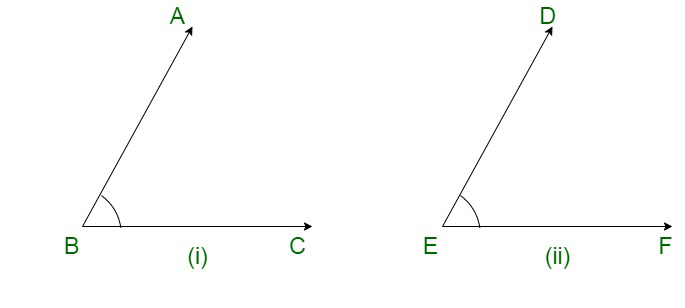Solution:

The figure is given as follows:

It is given that, arms BA and BC of ∠ABC are respectively parallel to arms ED and EF of ∠DEF..

We need to show that ∠ABC = ∠DEF

Let us extend BC to meet EF.

We have AB || DE ∠ABC and ∠DEF are corresponding angles, these two should be equal.

Therefore,

∠ABC = ∠DEF

Hence, proved.

### Question 25. In the given figure, arms BA and BC of ∠ABC are respectively parallel to arms ED and EF of ∠DEF. Prove that ∠ABC+ ∠DEF= 180°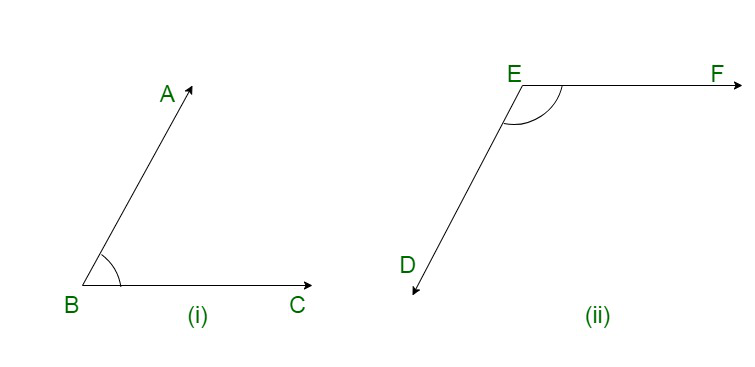Solution:

The figure is given as follows: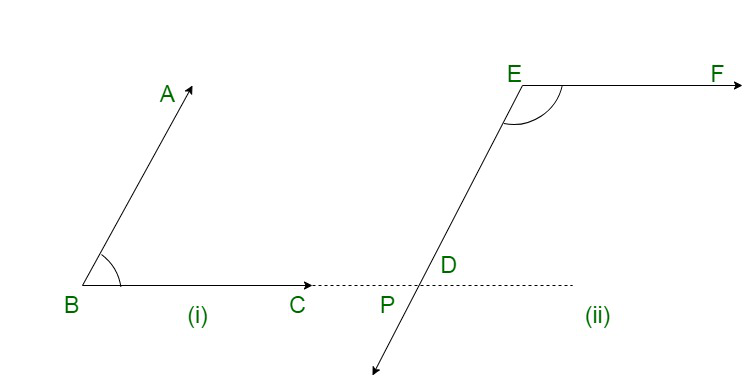It is given that, arms BA and BC of ∠ABC are respectively parallel to arms ED and EF of ∠DEF.

We need to show that ∠ABC + ∠DEF = 180°

Let us extend BC to meet ED at point P.

We have AB || DE and BP || EF. So, ∠BPE and ∠PEF are corresponding angles, these two should be equal.

Therefore,

∠BPE = ∠PEF

Also, we have AB || PE. So, ∠ABP and ∠BPE are consecutive interior angles, these two must be supplementary.

Therefore,

∠ABP + ∠BPE = 180°

∠ABC + ∠PEF = 180°

∠ABC + ∠DEF = 180°

Hence, proved.

### Question 26. Which of the following statements are true (T) and which are false (F)? Give reasons.

(i) If two lines are intersected by a transversal, then corresponding angles are equal.

(ii) If two parallel lines are intersected by a transversal, then alternate interior angles are equal.

(iii) Two lines perpendicular to the same line are perpendicular to each other.

(iv) Two line parallel to the same line are parallel to each other.

(v) If two parallel lines are intersected by a transversal, then the interior angles on the same side of the transversal are equal.

Solution:

(i) Statement: If two lines are intersected by a transversal, then corresponding angles are equal.

False

Reason: The above statement holds good if the lines are parallel only.

(ii) Statement: If two parallel lines are intersected by a transversal, then alternate interior angles are equal.

True

Reason: Letlandmare two parallel lines.

And transversaltintersectslandmmakinga two pair of alternate interior angles, ∠1, ∠2 and ∠3, ∠4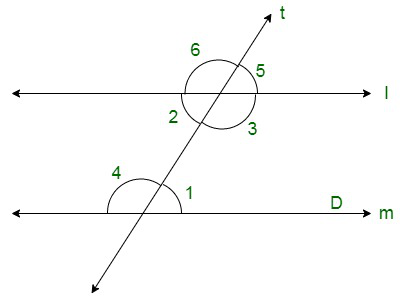We need to prove that ∠1 = ∠2 and ∠3 = ∠4.

We have,

∠2 = ∠5 (Vertically opposite angles)

And, ∠1 = ∠5 (corresponding angles)

Therefore,

∠1 = ∠2 (Vertically opposite angles)

Again, ∠3 = ∠6 (corresponding angles)

Hence, ∠1 = ∠2 and ∠3 = ∠4.

(iii) Statement: Two lines perpendicular to the same line are perpendicular to each other.

False

Reason:The figure can be drawn as follows: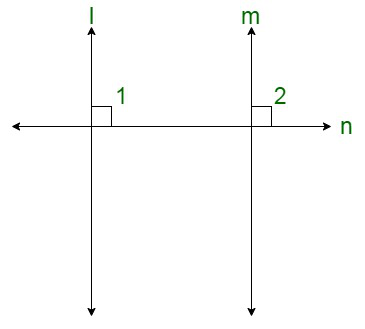Here, l ⊥ n and m ⊥ n

It is given that l ⊥ n, therefore,

∠1 = 90° …….(i)

Similarly, we have m ⊥n, therefore,

∠2 = 90° …….(ii)

From (i) and (ii), we get:

∠1 = ∠2

But these are the pair of corresponding angles.

Theorem states: If a transversal intersects two lines in such a way that a pair of corresponding angles is equal, then the two lines are parallel.

Thus, we can say that l || m.

(iv) Statement: Two lines parallel to the same line are parallel to each other.

True

Reason: The figure is given as follows: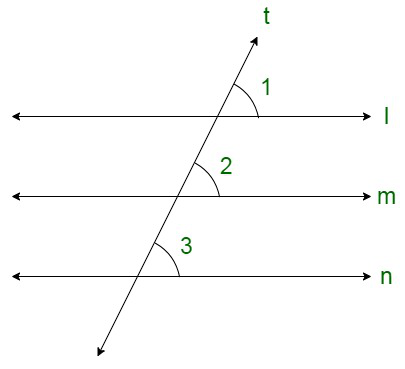It is given that l || m and m || n

We need to show that l || m

We have l || m, thus, corresponding angles should be equal.

That is,

∠1 = ∠2

Similarly,

∠3 = ∠2

Therefore,

∠1 = ∠3

But these are the pair of corresponding angles.

Therefore, l || m.

(v) Statement: If two parallel lines are intersected by a transversal, then interior angles on the same side of the transversal are equal.

False

Reason: Theorem states: If a transversal intersects two parallel lines then the pair of alternate interior angles is equal.

### Question 27. Fill in the blanks in each of the following to make the statement true:

(i) If two parallel lines are intersected by a transversal, then each pair of corresponding angles are …

(ii) If two parallel lines are intersected by a transversal, then interior angles on the same side of the transversal are ….

(iii) Two lines perpendicular to the same line are … to each other.

(iv) Two lines parallel to the same line are … to each other.

(v) If a transversal intersects a pair of lines in such away that a pair of alternate angles are equal, then the lines are …

(vi) If a transversal intersects a pair of lines in such away that the sum of interior angles on the same side of transversal is 180°, then the lines are …

Solution:

(i) If two parallel lines are intersected by a transversal, then corresponding angles are equal.

(ii) If two parallel lines are intersected by a transversal, then interior angles on the same side of the transversal are supplementary.

(iii) Two lines perpendicular to the same line are parallel to each other.

(iv) Two lines parallel to the same line are parallel to each other.

(v) If a transversal intersects a pair of lines in such a way that a pair of interior angles is equal, then the lines are parallel.

(vi) If a transversal intersects a pair of lines in such a way that a pair of interior angles on the same side of transversal is 180°, then the lines are parallel.

My Personal Notes arrow_drop_up
Related Tutorials